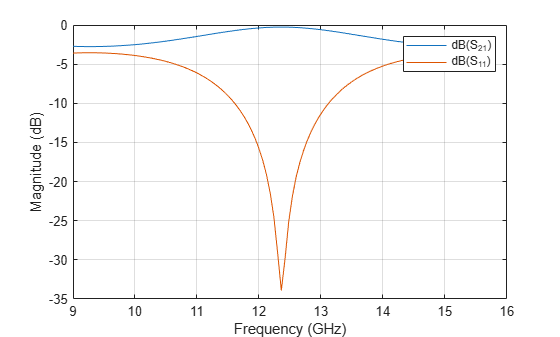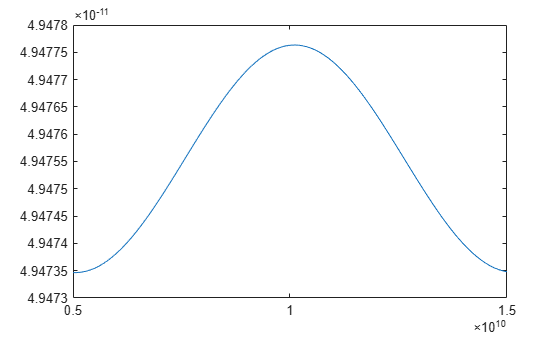# txlineStripline

Create stripline transmission line element

## Description

Use the `txlineStripline` object to create a stripline transmission line element. The cross-section of a stripline transmission line is shown in the following figure. The physical characteristics of a stripline transmission line include strip width w, strip length l, strip thickness t, substrate height h, and relative permittivity constant ε. You can also use the `txlineStripline` object to model a delay lossless transmission line in an RF system using the `rfbudget` object or the RF Budget Analyzer app.## Creation

### Syntax

``txline = txlineStripline``
``txline = txlineStripline(Name=Value)``

### Description

example

````txline = txlineStripline` creates a stripline transmission line object.```

example

````txline = txlineStripline(Name=Value)` sets properties using one or more name-value arguments. For example, ```txline = txlineStripline(Width=0.0046)``` creates a standard stripline transmission line with a width of 0.0046 meters. Properties you do not specify retain their default values.```

## Properties

expand all

Name of the stripline transmission line, specified as a string scalar or a character vector.

Example: `Name='stripline1'`

Physical width of the stripline transmission line, specified as a positive scalar in meters.

Example: `Width=0.0008`

Data Types: `double`

Physical thickness of the dielectric, specified as a positive scalar in meters.

Example: `DielectricThickness=0.00835`

Data Types: `double`

Physical thickness of the stripline transmission line, specified as a positive scalar in meters.

Example: `Thickness=0.00002`

Data Types: `double`

Relative permittivity of the dielectric, specified as a positive scalar.

Example: `EpsilonR=8.8`

Data Types: `double`

Loss angle tangent of the dielectric, specified as a nonegative scalar.

Example: `LossTangent=0.01`

Data Types: `double`

Conductivity of the conductor, specified as a nonnegative scalar in Siemens per meter (S/m).

Example: `SigmaCond=2`

Data Types: `double`

Physical length of the stripline transmission line, specified as a positive scalar in meters.

Example: `LineLength=0.0200`

Data Types: `double`

Stub transmission line termination, specified as either `'NotApplicable'`, `'Open'`, or `'Short'`.

Example: `Termination='Short'`

Data Types: `char`

Type of stub, specified as either `'NotAStub'`, `'Series'`, or `'Shunt'`.

Example: `StubMode='Series'`

Data Types: `char`

Number of input and output ports, returned as a positive scalar.

Data Types: `double`

Terminals of the stripline transmission line, returned as a cell array of strings.

Data Types: `char` | `string`

## Object Functions

 `sparameters` Calculate S-parameters for RF data, network, circuit, and matching network objects `groupdelay` Group delay of S-parameter object or RF filter object or RF Toolbox circuit object `noisefigure` Calculate noise figure of transmission lines, series RLC, and shunt RLC circuits `getZ0` Calculate characteristic impedance with and without dispersion for transmission line `circuit` Circuit object `clone` Create copy of existing circuit element or circuit object

## Examples

collapse all

Create a 10 GHz stripline transmission line.

``` stl = txlineStripline(Width=0.08e-3,DielectricThickness=1.6e-3,LineLength=12.2777e-3,... Thickness=0.01e-3,EpsilonR=3.9,SigmaConductivity=5.88e7);```

Calculate the S-parameters of the stripline transmission line.

``` freq = linspace(9e9,15e9,101); spar1 = sparameters(stl,freq,50);```

Plot the S21 and S11 of the stripline transmission line.

` rfplot(spar1,[2 1],1)`Create a stripline transmission line object.

` txline = txlineStripline;`

Calculate the group delay of the stripline transmission line.

```freq = linspace(5e9,15e9,101); gd = groupdelay(txline,freq,Impedance=50);```

Plot the group delay of the stripline transmission line.

`plot(freq,gd)`Calculate the characteristic impedance of the stripline transmission line.

`char = getZ0(txline,10e9)`
```char = 49.5426 ```

## Version History

Introduced in R2022a

expand all